Hydrol. Earth Syst. Sci., 24, 1189–1209, 2020
https://doi.org/10.5194/hess-24-1189-2020
Hydrol. Earth Syst. Sci., 24, 1189–1209, 2020
https://doi.org/10.5194/hess-24-1189-2020

Research article 12 Mar 2020

Research article | 12 Mar 2020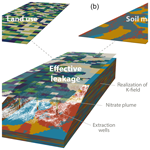# On the conceptual complexity of non-point source management: impact of spatial variability

On the conceptual complexity of non-point source management: impact of spatial variability
Christopher Vincent Henri1, Thomas Harter1, and Efstathios Diamantopoulos2 Christopher Vincent Henri et al.
• 1University of California, Davis, Center for Watershed Sciences, Veihmeyer Hall, Davis, CA 95616, USA
• 2University of Copenhagen, Department of Plant and Environmental Sciences, Thorvaldsensvej 40, Copenhagen, 1871, Denmark

Correspondence: Christopher Vincent Henri (chenri@ucdavis.edu)

Abstract

Non-point source (NPS) pollution has degraded groundwater quality of unconsolidated sedimentary basins over many decades. Properly conceptualizing NPS pollution from the well scale to the regional scale leads to complex and expensive numerical models: key controlling factors of NPS pollution – recharge rate, leakage of pollutants, and soil and aquifer hydraulic properties – are spatially and, for recharge and pollutant leakage, temporally variable. This leads to high uncertainty in predicting well pollution. On the other hand, concentration levels of some key NPS contaminants (salinity, nitrate) vary within a limited range (< 2 orders of magnitude), and significant mixing occurs across the aquifer profile along the most critical compliance surface: drinking water wells with their extended vertical screen length. Given these two unique NPS contamination conditions, we here investigate the degree to which NPS travel time to wells and the NPS source area associated with an individual well can be appropriately captured, for practical applications, when spatiotemporally variable recharge, contaminant leakage rates, or hydraulic conductivity are represented through a sub-regionally homogenized parametrization. We employ a Monte Carlo-based stochastic framework to assess the impact of model homogenization on key management metrics for NPS contamination. Results indicate that travel time distributions are relatively insensitive to the spatial variability of recharge and contaminant loading, while capture zone and contaminant time series exhibit some sensitivity to source variability. In contrast, homogenization of aquifer heterogeneity significantly affects the uncertainty assessment of travel times and capture zone delineation. Surprisingly, the statistics of relevant NPS well concentrations (fast and intermediate travel times) are fairly well reproduced by a series of equivalent homogeneous aquifers, highlighting the dominant role of NPS solute mixing along well screens.

Share
1 Introduction

The use of agrochemicals to address an ever-growing food demand has led to the contamination of many sedimentary groundwater basins underlying intensively farmed regions . Given the broad, continuous expanse of agricultural pollution sources across affected groundwater basins, this type of large-scale pollution is often referred to as non-point source (NPS) pollution . The development of effective protection or remediation strategies in groundwater bodies affected by NPS pollution will require understanding of the dynamics of such pollution in groundwater systems. Important aspects of NPS pollution are pollutant travel times, the location of well source areas (also known as capture zones; ) to identify specific pollution sources, and the long-term evolution of contaminant levels in and across affected wells and streams. The predictive modeling of these processes and associated management metrics is challenged by the inherent complexity of NPS pollution in groundwater systems.

Spatial variability represents a key source of complexity to be considered in understanding pollutant transport in the subsurface. Decades of investigation at contaminated industrial sites have highlighted the critical role that aquifer heterogeneity (e.g., the hydraulic conductivity) has in accurately understanding the solute transport behavior to identify polluters and to design effective remediation schemes and assess associated risk . Large aquifer heterogeneity significantly affects the macro-dispersive behavior of contaminant plumes emanating from point sources. Lacking data to characterize subsurface properties in sufficient detail introduces significant uncertainty in the prediction of solute transport, the design of remediation measures, and the prediction of future concentrations at wells of interest (e.g., Dagan1984; Rubin2003). The prediction of solute transport from the NPS to a compliance area of interest (e.g., extraction or observation wells) has been shown to be critically impacted by aquifer heterogeneity but also by mixing along the screen of production wells: contaminant mass arrivals in extraction wells may take decades to centuries and are characterized by significant uncertainty .

Unique to non-point sources, the spatial (and temporal) variability of the source itself across a groundwater basin introduces an additional level of system complexity. NPS pollution of groundwater is typically associated with dissolved solutes associated with groundwater recharge across the landscape. Both recharge rates and contaminant concentrations in non-point sources are subject to large spatial and temporal variability. The variability is partly due to spatially variable soil properties (e.g., Nielsen et al.1973). These properties control infiltration, recharge to groundwater, and the fate and transport of contaminants in the unsaturated zone (Hillel1980). Landscape management that leads to NPS pollution releases, e.g., irrigation, fertilization, construction and maintenance of urban, domestic, and other distributed waste systems leaking incidentally or intentionally into groundwater, is also subject to large spatial and temporal variability . As with aquifer properties, the minutia of such spatial and temporal variability cannot be measured (or estimated) except at larger scales. For example, to the degree that differences exist in average recharge and pollutant loading between mappable landscape management systems, these may be explicitly represented in space and time (e.g., Loague and Corwin1998; Nolan et al.2018). This includes NPS differences between different farming systems or between crops . Similarly, the degree to which mappable soil units affect recharge and pollutant fate and transport to the water table can also be explicitly represented . However, spatial variability at smaller spatial scales or between individual units of the same mappable class are subject to stochastic variability . Furthermore, both the timing and the spatial distribution of mappable and smaller-scale unknown landscape processes is a stochastic process from a regional management perspective, which is concerned with pollution dynamics across an ensemble of wells.

The dual complexity of aquifer heterogeneity and spatiotemporal source variability represent a largely unexplored challenge in the assessment and management of NPS pollution in aquifers. Yet, conceptually simplified approaches have been successfully employed to predict general trends and expected (average) contaminant behavior across ensembles of pollutant receptors of interest (wells, stream reaches) (e.g., Conan et al.2003). Typically, these assessments lack any measures to also assess predictive uncertainties.

Some key characteristics of NPS contamination on the other hand make the NPS pollution system in groundwater well suited for upscaling without loss of information relevant to understanding the range of impacts on receptors: first, the individual compliance surface of interest (the groundwater–well interface, the groundwater–stream reach interface) is subject to complete mixing prior to exposure (extracted well water, stream reach baseflow contribution). For example, production wells for urban water supplies are typically screened over dozens of meters . Even domestic wells are typically screened vertically over several meters of an aquifer system . Similarly, stream reaches mix across an aquifer area of several tens to tens of thousands of square meters. The source area associated with such significantly sized compliance surfaces typically has length scales exceeding 100 m and frequently exceeding 1 km . As a result, extracted water will be a mixture of groundwater age and source water quality .

Secondly, while the source is widespread, compliance levels of key NPS contaminants (e.g., salt, nitrate) are commonly much less than 1 order of magnitude lower than the concentration in NPS recharge. This is characteristically different from most point-source contamination, where concentrations at the source may exceed compliance levels by many orders of magnitude (e.g., Frind et al.1999). With the smaller difference between compliance and NPS recharge concentration, mixing at the compliance surface (i.e., in the well screen, at the stream reach scale) acts to homogenize the NPS recharge signature in both space and time, thus reducing the need to accurately characterize the variability in space or in time to determine the mixed concentration at an individual compliance surface . In contrast to assessing point sources of industrial pollution, significant simplification in the spatiotemporal representation of both or either water and contaminant leakage rates and hydraulic conductivity may be possible without loss of accuracy. explored the homogenization of temporal variability in NPS behavior. Yet little work has been done to better understand and quantify the degree to which spatiotemporal variability in NPS representation or spatial variability in aquifer representation can be homogenized in NPS simulation tools while still accurately predicting NPS management metrics, including concentrations at compliance surfaces.

In this paper, we assess the degree to which detailed spatial representation of both the aquifer hydraulic conductivity and of contaminant source parameters – recharge rate of water and contaminant loading from the NPS to the groundwater table – can be homogenized in NPS models without reducing model accuracy. We consider three NPS management metrics and use a comparative simulation approach for our assessment.

Our starting point is a set of simulations that predict the long-term contamination of an aquifer from NPS pollution under highly resolved heterogeneous aquifer and NPS source conditions. Results are compared against various simulation scenarios with homogenized representations of the aquifer and source heterogeneity. We compare results from various homogenization scenarios by focusing on three stochastic management metrics: the travel time distribution to production wells, the stochastic capture zone, and the stochastic contaminant time series in well water. Stochastic management metrics are quantified both for the pollution variability across an ensemble of production wells encountered over a basin and for the uncertainty about pollution levels at an individual well. The later assumes structural ergodicity (Dagan1990), i.e., that the mean and variance of a single realization of the hydraulic conductivity field are close to the same statistics of the ensemble distribution (see the histograms in Supplement Fig. S1).

2 Methodology

## 2.1 Reference case

We consider an unconsolidated sedimentary aquifer system typical of the Central Valley (California, USA), initially uncontaminated (e.g., pre-development state) and subject to nitrate pollution from agricultural NPS sources. The sub-region is characterized by a semi-arid Mediterranean climate, with dry summers and significant winter precipitation occurring mostly via the surrounding mountain ranges. The Central Valley groundwater basin is subject to intensive irrigated agricultural activities supported by reservoirs managing surface water inflows from surrounding mountain ranges and by groundwater. Over the past 8 decades, irrigation and groundwater pumping have added a significant vertical flow component: lateral groundwater flow fed by mountain front recharge and discharged along the thalweg used to dominate the groundwater system dynamic. Modern groundwater discharge is mostly due to groundwater extraction. Recharge from intensive irrigation is superimposed on a weak lateral gradient, significantly increasing the importance of downward flows (Faunt2009). Water recharged from the irrigated landscape to groundwater bodies carries significant loading of agricultural NPS pollutants, such as salt or nitrate (e.g., Baram et al.2016).

The simulated soil and aquifer contamination setting represents conditions typically encountered in the Central Valley’s agricultural basins, but are not specific to a particular location. We represent heterogeneity in the hydraulic conductivity as well as the spatial variability in soil types and land use. The latter two are key characteristics that control spatial variability in recharge and contaminant leakage rates. The transfer of water and nitrate from the soil surface to the aquifer is estimated through the modeling of flow and transport in the unsaturated zone for a series of typical soil types and crops found in the Central Valley.

### 2.1.1 Stochastic analysis

Uncertainty in the representation of the spatial variability of the aquifer and soil hydraulic conductivity is systematically accounted for through the use of a geostatistical model in a Monte Carlo framework (Rubin2003). The propagation of variability and uncertainty into management metrics is assessed across an ensemble of production wells. Assuming ergodicity (Dagan1990), stochastic analysis is applied to first quantify uncertainty about pollution outcomes at individual wells and to secondly quantify regional spatial variability in pollution outcomes across an ensemble of wells: to characterize the uncertainty at an individual well, a large number of realizations of individual wells with equiprobable aquifer and soil realizations is generated. Flow and transport processes across each are solved using a specified (fixed), mappable land-use representation. To assess the spatial variability of NPS management metrics across an ensemble of well locations in a groundwater basin, the equiprobable realizations of the aquifer system represent the variety of locations across a basin with geostatistically similar geological features. This is true since the domain is designed to ensure that source areas of the three production wells are fully accommodated and that each well's area of capture can be considered independent. In the case of a regional analysis, land use is simulated as a random process.

Then, stochastic management metrics quantify both the mean and variability of pollution levels across a large sample of production wells encountered over a basin as well as the expected value and uncertainty about pollution levels at an individual well. This is done by simulating stationary random fields (Fig. S3) and assuming ergodic conditions (e.g., ).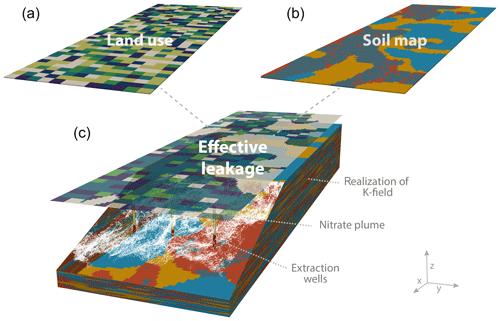Figure 1Illustration of the methodology used in the study, considering a representative quadrilateral subsystem of a highly heterogeneous alluvial aquifer system, $\mathrm{19.2}×\mathrm{6}×\mathrm{0.25}$ km3 (12 miles ×3.75 miles ×820 feet). A land-use map is randomly generated (a). Each color represents a different crop. A soil map (b) is extracted from the top layer of each geostatistical realization of the hydraulic conductivity (K). For each combination soil-type–crop, effective leakage rates of water and nitrate are numerically estimated. White particles represent a snapshot of the NPS pollution (particles) 80 years after a single year of contaminant loading. In each simulation, three extraction wells are explicitly represented at the downstream location of the domain. The lower part of each well (in red) represents its screen from where water is extracted and from where mass arrival is recorded. Other wells are implicitly represented by the flux into the lower boundary of the domain.

### 2.1.2 Aquifer

Spatial variability in the aquifer hydraulic conductivity (K) is represented using the transition probability/Markov chain method (Carle1999) for generating random realizations of the hydrofacies field . Here, we consider four hydrofacies: gravel, sand, muddy sand and mud. The geostatistical model requires the characterization of the proportion, mean length and hydraulic conductivity of each facies, and of the facies-to-facies transition probability rates. We set these parameters to be representative of Central Valley aquifer conditions (Table 1 and 2). A total of 50 realizations of the K field was generated. An example of a K field can be observed in Fig. 1. The histograms of the mean and the variance of the logarithm of K are shown in Fig. S3. Fifty realizations were sufficient to converge the lower statistical moments of K and of the resulting mean velocities (Fig. S9).

Table 1Proportion and hydraulic conductivity of the four categories (g: gravel; s: sand; ms: muddy sand; m: mud).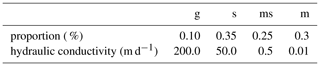Table 2Mean length (diagonal values) of each category (g: gravel; s: sand; ms: muddy sand; m: mud) and embedded transition probability (non-diagonal values) in the longitudinal (x), horizontal transverse (y), and vertical transverse (z) directions. Matrices read as transition probabilities from the row facies to the column facies. The background category is designated by the letter b.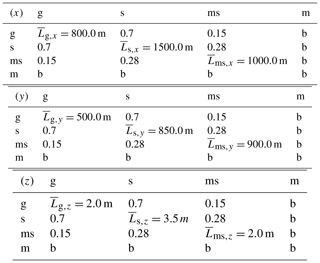### 2.1.3 Soil map

The top layer of each K-field realization is here considered to represent the (unmapped) spatial variability of the soil type. Thus, a soil map, displaying the spatial distribution of the four hydrofacies at the land surface, is geostatistically consistent with each realization of the aquifer K field underlying the soil horizon.

### 2.1.4 Land use

The landscape of the simulated sub-basin is considered to be exclusively occupied by agricultural activities. Six different crop types are randomly distributed over a domain of 19200 m ×6000 m. The crops are almond, citrus, corn, cotton, grain and grapes. All fields are of the same rectangular dimension, 360 m ×300 m (11 ha, 27 acres). The spatial distribution of crop types is generated randomly and fulfills the following proportions of the six crop types: 24 % of almond, 24 % of citrus, 18 % of corn, 12 % of cotton, 12 % of grain, and 10 % of grapes (Table 3). Crop types and proportion are representative of what may be encountered in the southeastern Central Valley .

### 2.1.5 Estimation of recharge and contaminant leakage

Numerical simulations were conducted to simulate the vadose zone flow and transport processes across all possible crop and soil-type combinations. Here, the gravel category in a soil layer was assumed to represent the same sandy soil as the sand category. Hence, a total of 18 vadose zone profiles represent all possible combinations of the 6 different land types (crops) and 3 different hydraulic soil profiles (sand, muddy sand, and mud). Simulations provide time-varying recharge and pollutant leakage rates for each of the 18 possible land-use and soil combinations at the water table of the respective underlying aquifer system. The time series of the 18 simulations are computed a priori and then applied to define the water table boundary conditions of the groundwater flow and transport simulations.

### Governing equations

One-dimensional water flow in soils is described by the Richards equation :

$\begin{array}{}\text{(1)}& \frac{\partial \mathit{\theta }}{\partial t}=\frac{\partial }{\partial z}\left[{K}^{\prime }\left(\mathit{\psi }\right)\left(\frac{\partial }{\partial z}+\mathrm{1}\right)\right]-S,\end{array}$

where θ (–) is the volumetric water content, t (d) is time, z (m) is the vertical spatial coordinate, positive upward, ψ (m) is the pressure head, K(ψ) (m2 d−1) is the saturated/unsaturated hydraulic conductivity as a function of ψ and S is the sink term, representing root water uptake (d−1). The water retention curve θ(ψ) and the hydraulic conductivity curve are required. The two functions are described by the van Genuchten–Mualem model:

$\begin{array}{}\text{(2)}& \mathit{\theta }\left(\mathit{\psi }\right)=\left\{\begin{array}{ll}{\mathit{\theta }}_{\mathrm{r}}+\left({\mathit{\theta }}_{\mathrm{s}}-{\mathit{\theta }}_{\mathrm{r}}\right)×\left(\mathrm{1}+\mid \mathit{\alpha }\mathit{\psi }{\mid }^{n}{\right)}^{-m}& \mathit{\psi }<\mathrm{0},\\ {\mathit{\theta }}_{\mathrm{s}}& \mathit{\psi }\ge \mathrm{0},\end{array}\right\\text{(3)}& {S}_{\mathrm{e}}=\frac{\mathit{\theta }\left(\mathit{\psi }\right)-{\mathit{\theta }}_{\mathrm{r}}}{{\mathit{\theta }}_{\mathrm{s}}-{\mathit{\theta }}_{\mathrm{r}}},\text{(4)}& {K}^{\prime }\left({S}_{\mathrm{e}}\right)={K}_{\mathrm{s}}×{S}_{\mathrm{e}}^{l}×{\left[\mathrm{1}-{\left(\mathrm{1}-{S}_{\mathrm{e}}^{\mathrm{1}/m}\right)}^{m}\right]}^{\mathrm{2}},\end{array}$

where θs and θr (–) are the saturated and residual water contents, respectively, α (m−1), n (–), m (–), and l (–) are shape parameters, $m=\mathrm{1}-\mathrm{1}/n,n>\mathrm{1}$, and Se (–) is the effective saturation.

Solute transport for a conservative tracer is described using a standard advection–dispersion equation of the form

$\begin{array}{}\text{(5)}& \frac{\partial \mathit{\theta }c}{\partial t}=\frac{\partial }{\partial z}\left(\mathit{\theta }D\frac{\partial c}{\partial z}\right)-\frac{\partial qc}{\partial z}-S×c,\end{array}$

where c (g m−3) is the concentration of the solute in the liquid phase, D (m2 d−1) is the dispersion coefficient, q is the volumetric water flux density (m d−1) evaluated with the flow equation and S×c (g m−3 d−1) is the root nutrient uptake for the case of passive uptake. By focusing on hydrodynamic dispersion, D is defined as

$\begin{array}{}\text{(6)}& D=\mathit{\lambda }q/\mathit{\theta },\end{array}$

where λ is the dispersivity (m).

### Parametrization of Hydrus 1D

For the numerical solutions of Eqs. (1) and (5), we used the Hydrus 1D software . Root water uptake for the six different crops was simulated by assuming a macroscopic root water uptake approach . The parameters for Eqs. (2) and (5) were estimated by using the Rosetta pedotransfer function and are shown in Table 4. For each soil horizon, dispersivity values were calculated by using the pedotransfer function of .

Table 3Area distribution, fertilization application and root zone depth for the five crops considered in this study.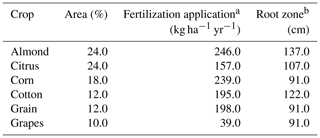a From . b From the .

Table 4Horizon depth, soil hydraulic properties and dispersity of each horizon, for the three different soil profiles assumed in this study.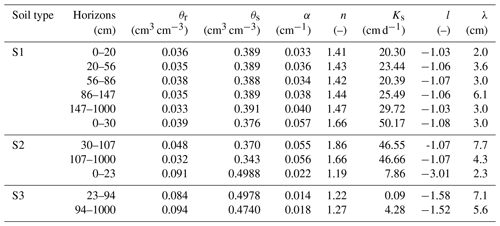The simulation time was 21 years, from 1 January 1995 until 31 December 2015. Of the 21 years, the first 11 were used as a warm-up period and the remaining 10 were used to represent temporally variable boundary conditions at the top of the groundwater system. For an initial condition of Eqs. (1) and (5), we assumed a uniform distribution of the pressure head and a solute-free profile, respectively. The upper boundary condition for the flow problem accounts for precipitation, irrigation, and crop evapotranspiration. Daily reference (grass) evapotranspiration (ET0) and precipitation (P) from the Stratford Meteorological Station (California Irrigation Management Information System (CIMIS)) are used to represent southeastern Central Valley climate conditions. For each crop, ET0 values were converted to potential crop evapotranspiration (ETc) by using the single crop coefficient method . Daily time series of boundary conditions are used in Hydrus 1D. Based on calculated ETc and P values, we created an irrigation schedule for each combination of crop-soil type, using the so-called evapotranspiration method . Irrigation was assumed to take place only during the crop period and not through the winter period (Fig. 2). For all crop–soil combinations, we assume three fertilization events per year with the total amount of fertilizer application given in Table 3.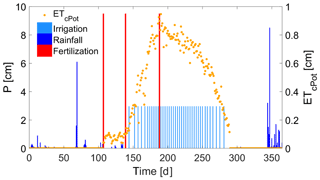Figure 2Illustration example of daily values of potential crop evapotranspiration (orange points) used for the description of the upper boundary condition. The blue bars represent rainfall events and the light blue bars irrigation events. The red bar defines fertilization application for an amount equal to 65 kg ha−1 (195 kg ha−1 yr−1). The crop is cotton.

### Preparing water table boundary conditions from unsaturated zone simulation results

Simulations led to an estimation of the temporal evolution of water and nitrate leakage rate at the bottom of the 1-D profile (Figs. S1 and S2) for each crop–soil-type combination, at daily time steps. For the sake of simplicity, our groundwater simulations are conducted for steady-state flow (but transient transport) conditions. Following , we homogenize both recharge and pollutant flux in time and compute average, effective recharge and nitrate leakage rates over a 10-year time series (illustration in Figs. S1 and S2 and average values in Table 5). For each of the 50 K-field (and, therefore, soil map) realizations, the temporally homogenized results for each crop–soil combination are then used to describe the spatial distribution of the effective recharge (r(x,y)) and nitrate mass flux (m0(x,y)). Histograms of the mean and the variance of recharge rate (r) and initial concentrations (c0) are shown in Figs. S4 and S5, respectively.

Table 5Recharge rate and nitrate mass flux applied for each crop–soil-type combination.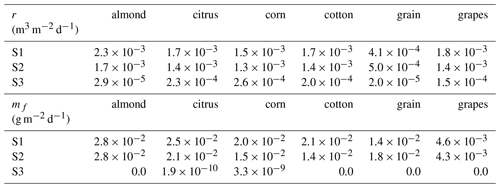## 2.2 Groundwater flow and transport

### 2.2.1 Flow

Groundwater flow and nitrate transport are numerically simulated. We consider a 3-D aquifer with a length (Lx) of 19200 m, a width (Ly) of 6000 m, and a depth (Lz) of 250 m (Table 6). Average steady-state groundwater flow conditions are governed by

$\begin{array}{}\text{(7)}& \frac{\partial }{\partial x}\left({K}_{xx}\frac{\partial h}{\partial x}\right)+\frac{\partial }{\partial y}\left({K}_{yy}\frac{\partial h}{\partial y}\right)+\frac{\partial }{\partial z}\left({K}_{zz}\frac{\partial h}{\partial z}\right)+{Q}_{\mathrm{s}}^{\prime }=\mathrm{0},\end{array}$

where h (m) is the hydraulic head, and ${Q}_{\mathrm{s}}^{\prime }$ is a volumetric flux per unit volume representing sources and sinks of water. Groundwater fluxes are simulated by solving numerically Darcy's law:

$\begin{array}{}\text{(8)}& \mathbf{q}\left(\mathbf{x}\right)=-K\left(\mathbf{x}\right)\mathrm{\nabla }h\left(\mathbf{x}\right),\end{array}$

where q (m d−1) is the specific discharge in the three dimensions $\mathbit{x}=\mathit{\left\{}x,y,z\mathit{\right\}}$. The longitudinal flow is defined by a regional gradient of $\mathrm{1.0}×{\mathrm{10}}^{-\mathrm{3}}$. The vertical flow is impacted by recharge and by a downward flux from the bottom of the domain. The spatially distributed fixed flux boundary condition across the bottom of the domain represents water flux to pumping wells in the deeper part of the aquifer and the effect of implied, non-simulated groundwater extraction by wells distributed throughout the lower part of the simulated aquifer sub-basin. Domestic wells are not considered to significantly affect flow and transport processes and are not simulated. Recharge is considered spatially variable to account for realistic spatial distribution of crop and soil types (see Sect. 2.1.5). For indications about the range of values and degree of variability, histograms of the mean and variance of the recharge rates applied in the 50 realizations of heterogeneous cases are shown in Fig. S4.

Table 6Domain discretization and physical parameters used in all the simulations.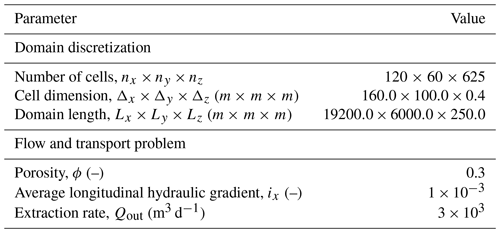Three extraction wells are located near the downstream edge of the domain. The extraction rate, Qout, is set to 3000 m3 d−1 (551 gpm), corresponding to an actual irrigation pumping rate of 6000 m3 d−1 (1102 gpm) or 9000 m3 d−1 (1653 gpm) during a 6-month or 4-month annual irrigation season, respectively. The length of the well screen is location and realization dependent, depending on the vertical distribution of highly conductive material (gravel, sand) (Appendix A). The total outflow (downward flux at the domain bottom plus extraction at the three wells) is set to be equal to the inflow of water by recharge. The resulting water flow system representation is solved using MODFLOW for each realization of the K field and the upper boundary.

### 2.2.2 Transport

$\begin{array}{}\text{(9)}& \mathit{\varphi }\frac{\partial c}{\partial t}=\mathrm{\nabla }\cdot \left(\mathit{\varphi }\mathbf{D}\mathrm{\nabla }c\right)+\mathrm{\nabla }\cdot \left(\mathbf{q}c\right),\end{array}$

where c is the solute concentration, D is the 3-D dispersion tensor, and ϕ is the porosity. The ADE is solved by the random walk particle tracking (RWPT) method implemented in Fortran code RW3D . RWPT solves the ADE by moving a large number of particles in successive jumps given by Salamon et al. (e.g., 2006).

$\begin{array}{}\text{(10)}& \begin{array}{rl}{\mathbf{x}}_{\mathrm{p}}\left(t+\mathrm{\Delta }t\right)& ={\mathbf{x}}_{\mathrm{p}}\left(t\right)+\mathrm{\Delta }t\left[\mathbf{v}\left({\mathbf{x}}_{\mathrm{p}}\left(t\right)\right)+\mathrm{\nabla }\cdot \mathbf{D}\left({\mathbf{x}}_{\mathrm{p}}\left(t\right)\right)\right]\\ & +\sqrt{\mathbf{D}\left({\mathbf{x}}_{\mathrm{p}}\left(t\right)\right)\mathrm{\Delta }t}\cdot \mathit{\xi }\left(t\right),\end{array}\end{array}$

where xp is the particle position, v is the velocity vector, and ξ is a normally distributed random variable with zero mean and unit variance.

The detailed discretization of the velocity field described above captures the most relevant characteristics affecting the macro-dispersive transport behavior . Therefore, effects of grid-scale dispersion are assumed to be negligible, i.e., D=0, and Eq. (10) is simplified to ${\mathbf{x}}_{\mathrm{p}}\left(t+\mathrm{\Delta }t\right)={\mathbf{x}}_{\mathrm{p}}\left(t\right)+\mathrm{\Delta }t×\mathbf{v}\left({\mathbf{x}}_{\mathrm{p}}\left(t\right)\right)$. This assumption, which potentially impacts NPS management metrics, is further evaluated in Appendix B.

Nitrate transport originating from the water table is simulated using an instantaneous injection of 500 000 particles over the entire top of the domain. Particle transport is tracked using the RWPT algorithm (Eq. 10) for a simulation time of 350 years. In simulations with spatially variable initial contaminant mass loading, the local density of particles reproduces the local initial concentration (c0(x,y)) in recharge water at the groundwater table. Histograms of the mean and variance of the initial concentration over the 50 realizations are shown in Fig. S5 if a visualization of the range of values and of the variability is needed. Following the superposition principle, the cumulative mass arrival at wells resulting from an instantaneous injection of mass m0 can be interpreted as $\stackrel{\mathrm{˙}}{m}\left(t\right)$, the simulated mass flux at wells resulting from a continuous and temporally constant release of mass m0. Well concentrations are computed as ${c}_{\mathrm{w}}\left(t\right)=\stackrel{\mathrm{˙}}{m}\left(t\right)/{Q}_{\mathrm{out}}$. Flow and transport are simulated for each realization of the K-field and water table boundary condition.

## 2.3 Non-point source pollution management metrics

Three relevant non-point source (NPS) pollution management metrics are considered to measure the stochastic simulation outcomes: the probability density function of pollutant travel times to wells, the probability distribution of pollutant concentration in wells, and the probability distribution of source locations.

### 2.3.1 Pollutant travel times

The probability density function of travel times is obtained by recording travel times of particles to the compliance area, that is, the screen of the extraction well, for each particle. We obtain normalized travel times ti by computing the time required to observe a specified fraction i of the total mass that reaches a well over the total 350-year simulation period. For instance, t5 represents the travel time from the water table to the well for the fifth percentile of the total mass reaching the well in 350 years. Following a stochastic approach, probability density functions (pdfs) of travel times ti are obtained using time series from 150 simulated wells (50 realizations, each with 3 wells). Figure S6 shows satisfactorily the convergence of the mean and variance of t50. Travel time pdfs can represent a useful tool to assess both the expected time of solute arrival at the compliance area and the propagation of uncertainty from the hydraulic conductivity field to pollutant transport .

### 2.3.2 Pollutant concentrations in wells (breakthrough curves)

The assessment of potential contaminant levels in extraction wells represents a key step in NPS pollution management. Under uncertain flow conditions, managers would benefit from knowing the probability of exceeding a threshold concentration such as the maximum contaminant level (MCL) in a given well or in a series of wells. For each of the 150 simulated wells, breakthrough curves c(t) are obtained. Figure S7 shows satisfactorily the convergence of the mean and variance of the concentration exceeded by 50 % of wells. Their probability distribution Pi(c,t) is obtained as a sample distribution of c(t), where Pi(c,t) is the probability of i % of wells exceeding the contaminant level c at time t.

### 2.3.3 Capture zones

NPS pollution management may also require the assessment of the effective source area, i.e., the capture zone or contributing area of the pollution observed in a production well. The spatial variability of hydraulic properties leads to uncertainty about and spatial variability of the source area . In the stochastic framework, the capture zone is assessed by defining the spatial distribution of the probability that a contaminant leaking from the NPS will reach a well (Pw), i.e.,

$\begin{array}{}\text{(11)}& \begin{array}{rl}{P}_{\mathrm{w}}\left({\mathbf{x}}_{\mathrm{NPS}}\right)& =\mathrm{Prob}\left({\mathbf{x}}_{\mathrm{p}}\left(t\in \left[\mathrm{0},{t}_{\mathrm{end}}\right]\right)\\ & ={\mathbf{x}}_{\mathrm{w}}\mid {\mathbf{x}}_{\mathrm{p}}\left(t=\mathrm{0}\right)={\mathbf{x}}_{\mathrm{NPS}}\right),\end{array}\end{array}$

where xp is the 3-D location (in the Cartesian coordinate system given by $\mathbit{x}=\left\{x,y,z\right\}$ of a portion of the plume (represented by a particle in this study), xw is a location shared with a well screen, and xNPS is a given location of the NPS. The spatial extension of non-zero probabilities then forms a probabilistic capture zone. The time required for a contaminant leaving a given location of the contributing area to reach the extraction well (or so-called time-related capture zone) is also stochastically analyzed.

3 Upscaling and test cases

## 3.1 Homogenization of source terms

The NPS metrics from fully heterogeneous simulations are compared to the NPS metrics obtained from a range of upscaled (e.g., Fleckenstein and Fogg2008), homogenized simulations that employ effective homogeneous properties rather than the original heterogenous distribution of the K, r, and c0 terms. The source terms (r(x,y), c0(x,y)) are homogenized separately for each realization by spatial averaging to obtain r and c0. Histograms of r and c0 show significant variability of the homogenized source terms between realizations (Figs. S3 and S4). Homogenized recharge rates and source concentrations range from 0.9 to 1.4 m d−1 m−2 and from 5.0 to 8.5 g m−3, respectively. A number of different homogenized models are considered and compared against the reference case:

• a heterogeneous r and heterogeneous c0 (reference case);

• a heterogeneous r and homogeneous c0;

• a homogeneous r and heterogeneous c0;

• a homogeneous r and homogeneous c0.

## 3.2 Homogenization of the hydraulic conductivity and transport upscaling

To simulate flow and transport in equivalent homogeneous, upscaled K conditions, we estimate the effective longitudinal and transverse vertical hydraulic conductivity, ${K}_{x}^{*}$ and ${K}_{z}^{*}$, and dispersion, ${\mathit{\alpha }}_{\mathrm{L}}^{*}$ and ${\mathit{\alpha }}_{\mathrm{TV}}^{*}$. The transverse horizontal (y-direction) component of transport is considered negligible given the size of the NPS plume and given that no gradient in y was applied. Effective parameters in the longitudinal direction (${K}_{x}^{*}$ and ${\mathit{\alpha }}_{\mathrm{L}}^{*}$) are determined from the first and second spatial moments of a plume resulting from an injection of mass in a vertical plane of width 3000 m and depth 50 m. The same approach is adopted to estimate the effective parameters in the transverse vertical direction (${K}_{z}^{*}$ and ${\mathit{\alpha }}_{\mathrm{TV}}^{*}$) by injecting particles in a horizontal plane covering the entire top of the domain. No extraction is considered in both cases in order to capture the natural behavior of the plume. For each realization of the K field, the slope of the temporal evolution of the first spatial moment, i.e., the plume center of mass, is used to evaluate the apparent velocities, ${v}_{x}^{*}$ and ${v}_{z}^{*}$. After estimation of the gradients from simulated head differentials in the x and z directions, Darcy's law is applied to evaluate effective hydraulic conductivities ${K}_{x}^{*}$ and ${K}_{z}^{*}$ (Eq. 8). Effective dispersion values (${\mathit{\alpha }}_{\mathrm{L}}^{*}$ and ${\mathit{\alpha }}_{\mathrm{TV}}^{*}$) are similarly obtained by analyzing the slope of the normalized second spatial moment of the particle plume. The importance of representing upscaled dispersion is independently tested. Histograms of results of upscaled ${K}_{x}^{*}$, ${K}_{z}^{*}$, ${\mathit{\alpha }}_{\mathrm{L}}^{*}$, and ${\mathit{\alpha }}_{\mathrm{TV}}^{*}$ values as well as the satisfactory convergence of the mean of the apparent parameters after 50 realizations are shown in Figs. S8 and S9, respectively.

Furthermore, the cumulative implication of homogenization in aquifer properties and in the source terms c0 and r is tested. The series of scenarios considered are

• a heterogeneous K, a heterogeneous r and a heterogeneous c0 (reference case);

• an upscaled K, r and c0, considering advection only;

• an upscaled K, a heterogeneous r and c0, considering advection only;

• an upscaled K, a heterogeneous r and c0, considering advection and upscaled dispersion.

4 Results and discussion: homogenization of source terms

The effect of conceptually simplifying recharge, contaminant input concentration, and aquifer heterogeneity on the stochastic description of travel times, well concentrations, and capture zones is here illustrated specifically for the case of quantifying uncertainty about these NPS pollution management metrics at a particular well surrounded by a spatially distributed but fixed (known) distribution of land use across all realizations (scenario “LU 1”). Alternatively, the effects of homogenization on the analysis of spatial variability across an ensemble of wells in a groundwater basin, where land use is different in each realization (scenario “LU 50”), are further discussed in Sect. 6.

## 4.1 Travel time

We analyze the pdfs of travel times for the fifth percentile (t5), 50th percentile (t50) and 95th percentile (t95) masses reaching a well within the 350-year simulation period (Fig. 3). These metrics characterize the temporal variability of the early, median, and late mass travel times from 1-year pollutant (e.g., nitrate) input to the aquifer system. For all simulations, early mass travel times are within a range of 10 to 100 years with a peak of probability of 50 years (Fig. 3a). Late mass travel times are likely to be in the range of 50 to 300 years (Fig. 3c), with a peak probability at about 120 years. These results are roughly consistent with the estimation of groundwater age distribution made by in the San Joaquin Valley from detailed modeling and from chlorofluorocarbon (CFC) age data (mean groundwater ages of 10 to 50 years in 2 to 3 times shallower wells than the ones simulated in this study).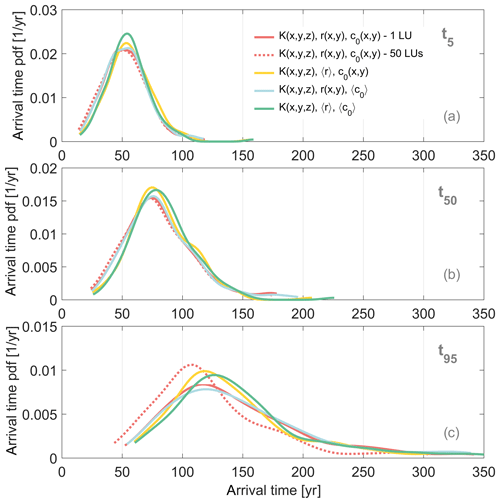Figure 3Probability density function of the time required for 5 % (a), 50 % (b), and 95 % (c) of the total recorded mass to reach a well considering a heterogeneous r and heterogeneous c0 (reference case, red line); a homogeneous r and heterogeneous c0 (yellow line); a heterogeneous r and homogeneous c0 (light blue line); a homogeneous r and homogeneous c0 (green line). Plain lines refer to the consideration of an identical land-use map for all realizations. For comparison, the red dashed lines show outputs from simulations with realization-dependent land-use maps.

The homogenization of recharge spatial variability directly affects the flow field in the uppermost part of the aquifer. While the effect is larger than the homogenization of the concentration (see the next paragraph), it also has no significant impact on travel time pdfs (Fig. 3): the distributions are slightly less spread out over the time axis, with slightly higher and earlier modes (peak of the pdf) and lower probabilities in the tails of the pdf (probability differences at all times < 5 %), especially of the late travel time (probability differences at all times around 10 %). Previously, investigated the impact of recharge spatial variability in a more theoretical and simplified 2-D heterogeneous aquifer contaminated by a point source under non-pumping conditions. The work highlights that spatial variability in recharge increases spreading, especially in the transverse direction. In our 3-D NPS setting, transverse spreading is less relevant (Fig. 3), and we do not observe the increase in variability.

The homogenization of initial concentrations has no physical impact on travel times since it does not affect the velocity field in the groundwater system. However, the difference in input concentration changes the distribution of the initial mass across the water table. Hence, there are small but discernible differences in the travel time pdfs of variable and homogeneous c both in the case of spatially variable r (red and blue lines in Figs. 3) and in the case of homogeneous r (yellow and green lines in Fig. 3).

Importantly, the homogenized representation of r and c has nearly no effect on the time span between early and late arrival times at the well screen (the contrast in the position of the travel time pdfs for t5 and t95), which represents the age difference between the youngest and oldest water captured by the well screen and then mixed during the pumping process .

## 4.2 Stochastic capture zone

The stochastic capture zone (or source area) is the area characterized by ${P}_{\mathrm{w}}\left(x,y\right)>\mathrm{0}$. Simulation results for the fully stochastic representation of source heterogeneity show that the stochastic capture zone covers an area of about 8000 by 4000 m (about 30 % of the simulated domain, containing approximately 300 individual fields), while the zone from where mass is most likely to reach a well (critical zone, ${P}_{\mathrm{w}}\left(x,y\right)>\mathrm{0.5}$) is more spatially focused (about 3 % of the simulated domain, the size of about 30 individual fields; see Fig. 4).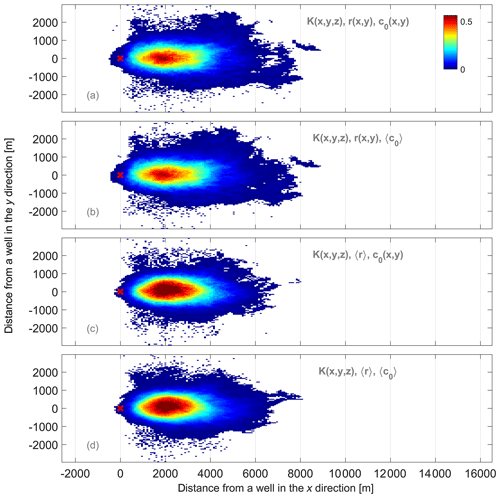Figure 4Probability of a particle leaving a given grid cell to reach a well accounting for a heterogeneous r and heterogeneous c0 (reference case, a); a heterogeneous r and homogeneous c0 (b); a homogeneous r and heterogeneous c0 (c); a homogeneous r and homogeneous c0 (d); a lnK-weighted r and lnK-weighted c0 (e).

As explained above, homogenizing the input concentration does not affect the velocity field and transport processes and only slightly reduces Pw values of the critical zone (Fig. 4b). On the other hand, not accounting for spatial variability in recharge leads to an overestimation of Pw values inside the critical zone (Fig. 4c). The same observation is made when both r and c0 are considered homogeneous (Fig. 4d). The location of the critical zone, being controlled by regional flow conditions and well characteristics (extraction rate, depth and length of the screen), is not impacted by the spatial description of source terms. Spatial variability in the recharge is responsible for somewhat more uncertainty (i.e., a decrease in the highest Pw values) in the exact delineation of the capture zone along its margins than what is captured by the homogenization of c0.

Recharge rates, if considered heterogeneous, are by design correlated with the hydraulic conductivity. Highly conductive material is associated with high recharge rates, which may increase the channeling effect through preferential paths. Accounting for spatial variability in the recharge rate will, therefore, exacerbate the impact of the heterogeneity on the K field, especially near the water table (where recharge is applied), thus increasing the uncertainty about delineating the source area.

Just as travel time pdfs are little affected (Fig. 3), the overall location of the stochastic capture zone is approximated quite well with the homogenized parametrization of concentration and recharge. Consequently, the average travel times required for a particle leaving a given location of the NPS to reach a well also are not dramatically impacted by the spatial representation of the two source terms (Fig. 5). The average flow condition is common to all simulations. Since the top of well screens is 100 m deep, the solute transport from the source to the compliance areas occurs mostly at depths far away from the spatially variable top boundary condition, where local changes in flow conditions at the surface does not impact significantly groundwater fluxes.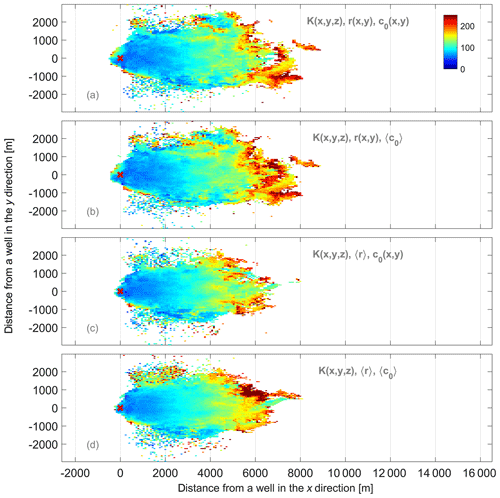Figure 5Expected travel time (years) of a particle leaving a given grid cell and reaching a well for a heterogeneous r and heterogeneous c0 (reference case, a); a heterogeneous r and homogeneous c0 (b); a homogeneous r and heterogeneous c0 (c); a homogeneous r and homogeneous c0 (d); a lnK-weighted r and lnK-weighted c0 (e).

## 4.3 Well NPS pollution concentration

A common characteristic of NPS pollution different from many point source cases is the temporal continuity and consistency in NPS inputs. For example, significant nitrate loading to groundwater began with the introduction of commercial fertilizer just before World War II and has continued since then . The long-term consequence of such continuous NPS loading, year after year, can be obtained from our simulations by superpositioning breakthrough curves obtained for NPS output in a single year at t=0. If we neglect long-term trends or year-to-year variations in NPS and assume a constant input of nitrate to the water table, then the stochastic breakthrough curve at the well screen is simply obtained by computing the cumulative distribution function (CDF) of the concentration pdf (Fig. 6). The CDF plots provide a measure of the expected time at which a given threshold contaminant level (in the x axis) such as the MCL (10 mg L−1 for nitrate as nitrogen in the US) will be exceeded with a probability of 90 % (P90, left), 50 % (P50, center), or 10 % (P10, right). In a regional context, these graphs can be interpreted as the time (x axis) after which at least 90 %, 50 %, and 10 % of all wells in the aquifer region exceed a concentration of interest (y axis), respectively.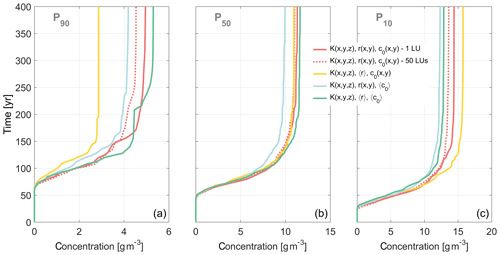Figure 6Time (y axis) required for a well to exceed a given concentration (x axis) with a probability of 90 % (a), 50 % (b) and 10 % (c) considering a heterogeneous r and heterogeneous c0 (reference case, red line); a homogeneous r and heterogeneous c0 (yellow line); a heterogeneous r and homogeneous c0 (light blue line); and a homogeneous r and homogeneous c0 (green line). For comparison, the red dash lines show outputs from simulations with a realization-dependent land-use map.

For the reference scenario (red curve in Fig. 6), we observe that the concentration eventually exceeded by 90 % of wells is about 4.5 mg N L−1, after about 250 years (left graph in Fig. 6). Half of the wells will have a concentration exceeding 11 mg N L−1 (again, after about 250 years). Also in at least half of the wells, the onset of rising nitrate levels (to above background levels) will occur no later than 50 years after the start of nitrate loading, reaching levels corresponding to half of the MCL (5 mg N L−1) after about 70 years, and reaching the MCL (10 mg N L−1) no later than about 150 years (middle graph in Fig. 6). The 10 % most nitrate contaminated wells will show an onset of nitrate contamination no later than 30 years after the start of NPS pollution, exceed the MCL in less than 70 years, and reach concentrations exceeding 14.5 mg N L−1 no later than about 150 years (right graph in Fig. 6).

For an individual well, the results indicate that there is a 10 % chance of nitrate concentrations starting to rise before 30 years, a 50 % chance of rising no later than 50 years, and a 90 % chance of rising before 70 years. Similarly, results suggest that the MCL will be exceeded with 10 % probability after 70 years and with 50 % probability after 140 years.

These results are consistent with observations of nitrate concentrations in drinking water and irrigation wells in the San Joaquin Valley, the southern half of the Central Valley. In Merced, Stanislaus, Tulare, and Kings County, about 40 % of domestic wells (with screen depths not exceeding 100 m) exceed the drinking water standard , but only about 10 % of the large production wells in the southeastern San Joaquin Valley (the wells represented in this study) exceed the nitrate MCL (10 mg N L−1) , approximately 70 years after the beginning of extensive fertilizer use in the region. We note that the timescale for these concentration increases is very sensitive to two aquifer parameters: the hydraulic conductivity and the average effective porosity. If the regional average K was twice as large as assumed in our model, all times would be half as long. Similarly, if the average regional effective aquifer porosity was 20 % larger, travel times would be 20 % shorter.

The homogenization of spatial variability in the recharge rate and in the source concentration, while of limited consequence to travel time estimates and to estimates of source area extent, has measurable implications for stochastic well concentration predictions, particularly at the lower margin: homogenizing only the recharge rate leads to significantly (>40 %) underestimating the maximum concentration exceeded by 90 % of wells in the intermediate and long term. Homogenization leads to somewhat (≈10 %) overestimating the concentration exceeded by 10 % of wells over the long term, but reproduces well the concentration exceeded by 50 % of wells at all times (yellow vs. red in Fig. 6).

Homogenizing both recharge and contaminant loading does not affect the predictions quite as much, and in the opposite direction: the (lower) concentrations exceeded by 90 % of wells are overestimated and the (higher) concentrations exceeded by only 10 % of wells are underestimated, while the concentrations exceeded by 50 % of wells are less than 5 % different from the fully stochastic prediction.

stochastically analyze the impact of spatially random recharge rate on transport in a 2-D point source setting. Their work concluded that, for those conditions, large variability in – and therefore uncertainty about – recharge increases uncertainty in solute concentration. In our work, we observe the opposite. The difference may be partly due to the 3-D non-point source transport and partly caused by the implicit correlation between the hydraulic conductivity and the recharge rate in our scenarios, which may increase the conditioning of the flow field that leads to the observed decrease in uncertainty relative to the homogenized scenario.

Results are also sensitive to a homogenization of only the initial concentrations, which would underestimate all concentrations by about 10 % (blue lines in Fig. 6). Homogenizing only concentration also leads to an underprediction, by about 20 %, of concentrations exceeded by either 90 %, 50 %, or 10 % of wells, relative to the fully stochastic land-use treatment (compare the blue and red lines in Fig. 6).

The results of the homogenization and the differences in treating land use in fully stochastic mode (“50 LUs”) are driven directly by three factors: the distribution of land use, including the sizes of fields relative to the source area, and the distribution of recharge and nitrate leaching among different land uses. As shown in Sect. 4.2, the extent of the capture zone encompasses hundreds of fields, while the critical capture zone – the core contribution area – encompasses at least 30 fields. For field sizes much larger than those simulated here or for a more spatially correlated distribution of crops among fields, homogenization across all land uses in a basin may lead to larger errors due to the smaller number of land-use “samples” intersected by the capture zone (Gibbons1994). Furthermore, unsaturated zone flow and transport simulations have led to the highest contaminant leakage rates in areas of high recharge (almonds, citrus; see Table 5). Homogenizing mass leakage therefore decreases the amount of contaminants in high recharge areas and consequently globally underestimates well concentrations. Outcomes would be different if the highest concentration is associated with the lower recharge rate.

The examples shown here indicate that there may be significant errors in predicting future concentrations exceeded by 90 % of wells and by 10 % of wells, i.e., the distribution of exceedance probabilities among the ensemble of wells, whereas the concentration exceeded by half of the wells is characterized quite accurately under homogenized land-use treatment. Overall, the homogenization of recharge in particular leads to the largest potential errors of NPS pollution management metrics, less so for predicting travel times and capture zone, but significantly so for predicting the distribution of exceedance concentrations across an ensemble of wells.

5 Results and discussion: homogenization of K

## 5.1 Travel time

In a second step, the implications of upscaling aquifer heterogeneity for the stochastic description of travel times, capture zones and well concentrations are assessed. Probability density functions of early, medium and late travel times are significantly impacted by the full homogenization of the hydraulic conductivity field (Fig. 7). A homogenization of both aquifer and land-use random processes (K, r, c0) drastically reduces the spread of all mass percentile travel times (yellow lines in Fig. 7). But the homogenized prediction of modes is quite accurate: the modes of the early mass and median mass travel times (t5 and t50) are predicted with about 10 % accuracy relative to the fully stochastic solution (Fig. 7a and b). For the late mass travel times, the mode in the homogenized prediction occurs later than for the case of a fully heterogeneous system (Fig. 7c).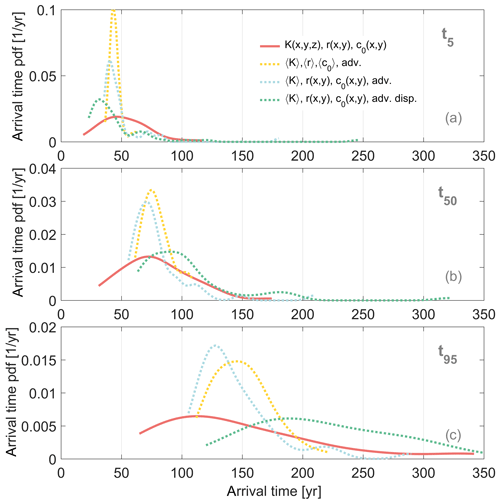Figure 7Probability density function of the time required for 5 % (a), 50 % (b), and 95 % (c) of the total recorded mass to reach a well considering a heterogeneous K field, heterogeneous r and heterogeneous c0 (reference case, red solid line); an upscaled K value, averaged r and averaged c0 accounting for advection only (yellow dashed line); an upscaled K value, heterogeneous r and heterogeneous c0 accounting for advection only (blue dashed line); an upscaled K value, heterogeneous r and heterogeneous c0 accounting for advection and dispersion (green dashed line).

Aquifer heterogeneity generates a complex network of well-connected channels but also zones of near stagnation, all of which controls the spatiotemporal behavior of contaminant plumes across all scales. The effective solute path architecture is, therefore, specific to the K-field realization and highly uncertain. In the stochastic solution, this generates a large range of probable solute travel times (travel time pdfs with large variance) to the well screen that cannot be captured by simulating transport in a homogenized K architecture (Fig. 7).

However, the global motion of the plume, characterized by its first spatial moment and the downward movement of the first moment along the depth interval of the well screen over time, would be approximately similar for all realizations given the geostatistical parameters and regional gradients. Thus, accounting for upscaled advective motion only (obtained from the estimation of the first spatial moment) preserves the large mixing in the well screen (Fig. A1) but underestimates the uncertainty in travel times arising from the macro-dispersive effects of heterogeneity. This is captured by the fact that the modes of the early, median, and late mass arrivals are spread over similar time periods (45 to 140 years); even the prediction based on a completely homogenized representation of both aquifer and land-use processes captures a significant fraction of the age distribution of mass arriving at the well screen. This is due to the significant mixing that occurs in the well screen when the well is being pumped .

Similar results to those for a fully homogenized representation are found when only the K field is homogenized, but land use is represented with heterogeneous r and c0. The spread of each mass percentile travel time pdf is slightly larger than in the fully homogenized case, but is relatively far from capturing the full extent of the travel time pdfs for the fully heterogeneous simulations (compare the blue and red lines in Fig. 7).

While the homogenization of K removes the controlling process of the macro-dispersive pollutant behavior, the macro-dispersive behavior can be approximated by including an upscaled, homogenized dispersion process (Eq. 9) in the simulation (Eq. 10). Using both homogenized K and a representative, effective macro-dispersion much improves the accuracy of the homogenized prediction and captures significant features of the fully stochastic prediction (green lines in Fig. 7). Early mass travel times are slightly underestimated, while median and especially late mass travel times are slightly overestimated.

Applying second spatial moments from heterogeneous simulations to estimate the macrodispersion of an upscaled homogeneous model, however, assumes that the macro-dispersion process follows a Gaussian process (Dagan1990). It has been shown here and in other work (e.g., Dagan1984; Cvetkovic et al.1992) that solute transport in heterogeneous media instead produces significantly skewed plume distributions, with early peak of mass and a long tail. Approximating such a skewed distribution with a Gaussian curve that is located at the same center of mass travel time and has the same second spatial moment is known to generate earlier first travel times, a later peak of mass, and later late travel times, consistent with our results. This complexity of upscaling transport from heterogeneous conditions to a simplified homogeneous aquifer using lower spatial moments only has been highlighted before. The results presented here confirm this observation for the case of non-point source contaminations but also highlight the generation of a quasi-macro-dispersive process through the (vertical) well mixing process.

## 5.2 Time-related capture zone

Analogous to the travel time pdfs, the spatial distribution of the stochastic capture zone, i.e., probability of a particle leaving a given location of the NPS to reach a well, is highly impacted by the homogenization of the hydraulic conductivity, much more so than by homogenization of land-use processes alone (Fig. 8).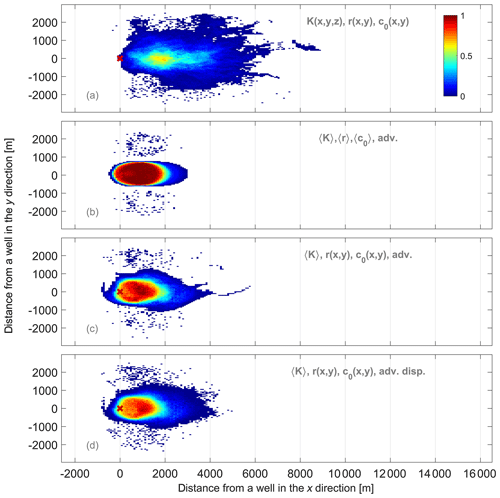Figure 8Probability of a particle leaving a given grid cell to reach a well accounting for a heterogeneous K field, heterogeneous r and heterogeneous c0 (reference case, a); an upscaled K value, averaged r and averaged c0 accounting for advection only (b); an upscaled K value, heterogeneous r and heterogeneous c0 accounting for advection only (c); an upscaled K value, heterogeneous r and heterogeneous c0 accounting for advection and dispersion (d).

In fully heterogeneous conditions, a wide range of Pw values are distributed over a large portion of the domain surface. However, most of the probabilistic capture zone is characterized by very low Pw values. The critical zone (area of highest probability) is characterized by Pw values of ≈0.6 and is centered at a longitudinal distance of about ≈2000 m from the well. In the solution to the equivalent homogeneous parameter and boundary conditions, the uncertainty of the capture zone location is significantly underestimated, with most of the capture zone being characterized by a high probability of reaching a well (Fig. 8b). Describing the spatial variability of nitrate mass loading and recharge (with a homogeneous K field) only adds a moderate degree of uncertainty to the capture zone delineation (i.e., lower highest Pw values), as expected from the travel time pdf results above. Utilizing the alternative homogenized transport modeling approach with a homogenized K and an equivalent macro-dispersion term, unlike for travel time pdfs, does not substantially improve the stochastic prediction of the capture zone (compare Fig. 8c and d). Furthermore, homogenizing the hydraulic conductivity seems, independently of the description of the source terms or of the consideration of dispersion, to mispredict the location of the capture zone: the critical zone is slightly moved downstream, closer to the wells, and the capture zone extends to a small portion of the downstream edge of wells. The most distant part of the critical zone in the homogenized prediction of the capture zone overlaps with the actual location of the critical zone in the fully stochastic solution.

Simulation outcomes highlight that the set of upscaled K values among the 50 realizations does not cover a range large enough to reproduce the high variability of original contaminant location expected in heterogeneous situation. This indicates that regional hydraulic vertical and longitudinal gradients, common to all simulations, control mostly the behavior of first spatial moments of heterogeneous plumes used here to estimate apparent velocities. Thus, contaminant mass reaching the top of the well has little variability – here only to the degree that the homogenization is done individually for each realization – leading to some minor realization-to-realization variability at the downstream side of the capture zone for the homogenized K (Fig. 8). More uncertainty is observed on the upstream side of the capture zone since it represents mass reaching the bottom of the screen, the vertical position of which is realization dependent.

Interestingly, the critical zone (high Pw) is predicted to be more downstream than its actual location if K is homogenized using apparent velocities (Fig. 8). In the case of heterogeneous K, a strong layering effect is observed, due to the superposition of relatively thin layers of highly and poorly conductive materials that stretch the plume longitudinally at large scale and move the capture zone upstream.

Consistent with these results, the spatial distribution of the mean travel time required for the contaminant to reach a well is similarly contracted to a much smaller area that extends downstream from the well, unlike in the fully stochastic representation (Fig. 9). The observed spatial variation of the mean travel times, increasing with the distance from a well, is overestimated when K fields are homogenized. This leads to higher predicted mean travel times over the entire capture zone for all tested aquifer simplifications.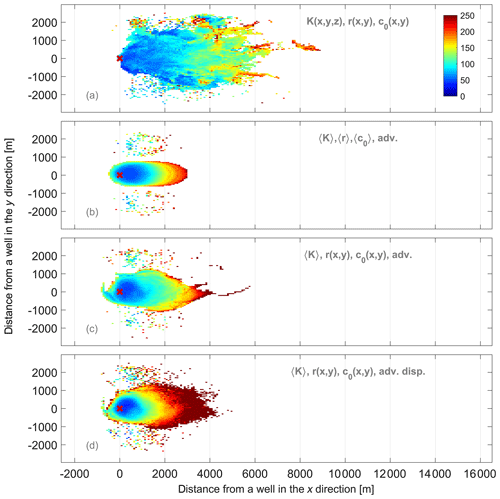Figure 9Expected travel time (years) of a particle leaving a given grid cell and reaching a well for a heterogeneous K field, heterogeneous r and heterogeneous c0 (reference case, a); an upscaled K value, averaged r and averaged c0 accounting for advection only (b); an upscaled K value, heterogeneous r and heterogeneous c0 accounting for advection only (c); an upscaled K value, heterogeneous r and heterogeneous c0 accounting for advection and dispersion (d).

## 5.3 Contaminant levels

Future concentrations exceeded by only 10 % of wells (P10) and those exceeded by half of the wells (P50) are captured to within a factor 2 for the transition period between 20 and 150 years, but agree to within 10 % with the fully stochastic simulation results at late times under near-steady-state pollution conditions. The (low) concentration levels exceeded by 90 % of wells (P90) differ by a factor 2 or more, at all times, from the fully stochastic solution (Fig. 10). Representing the spatial variability of source terms, but using a homogenized K field, improves the prediction of the P90 evolution.

Using the alternative homogenized representation with an equivalent macro-dispersion improves the prediction only at late time (>150 years) and predicts long-term concentrations for P50 and P10 very accurately (green lines in Fig. 10). But it underestimates the concentrations for all of P90 and during the transition time for P50 and P90.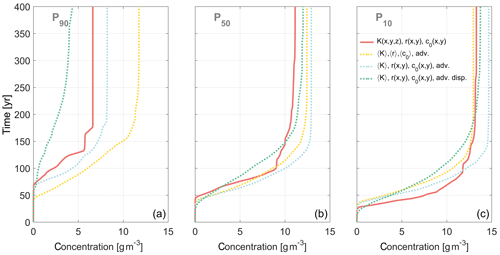Figure 10Time (y axis) required for a well to exceed a given concentration (x axis) with a probability of 90 % (a), 50 % (b) and 10 % (c) considering a heterogeneous K field, heterogeneous r and heterogeneous c0 (reference case, red solid line); an upscaled K value, averaged r and averaged c0 accounting for advection only (yellow dashed line); an upscaled K value, heterogeneous r and heterogeneous c0 accounting for advection only (blue dashed line); an upscaled K value, heterogeneous r and heterogeneous c0 accounting for advection and dispersion (green dashed line).

The agreement between fully stochastic solutions and the homogenized solutions is in contrast to the seemingly significant differences between homogenized and fully stochastic results observed for travel time distributions of the individual mass percentiles and the capture zone location. That the homogenized prediction is still capable of producing useful results is due to the unique properties of the non-point source pollution listed earlier: first, the NPS pollution is a continuous process rather than a one-time event, with some interannual variability and slow long-term trends . Second, the mixing of water quality occurring in the well screen greatly controls the observed pollutant levels because of the continuous loading and because differences in pollutant loading rates for the more permeable soils, across all crops (Table 5), vary within less than 1 order of magnitude. Third, the composition of the land use and therefore the recharge and mass loading rates vary at a scale that is much smaller than the source area of the well. Hence, any location of the source area will capture a similar overall mass of the NPS pollutant over time. Third, the amount of water quality mixing in the well is strongly controlled by the vertical location and length of the well screen and, for typical municipal production wells or agricultural wells, as simulated here, well construction will dominate the range of travel time distributions of water and solutes entering the well screen over effects of macro-dispersion. Reproducing the range of average regional gradients potentially observed in a region and average loading therefore provides critical and important information to reproduce in-well mixing of age and, hence, recorded water quality.

6 Results and discussion: regional stochastic analysis

Results and discussion thus far have focused on the uncertainty about predicting concentrations and source area associated with a single well, where land-use distribution is heterogeneous but deterministic (mappable). In the simulations discussed, the land use (but not the soil) was the same across all realizations. In NPS pollution management, an understanding of the variability in concentration evolution over time across the ensemble of wells in a basin, region, or management zone is of equal or more importance than understanding the uncertainty of future pollution dynamics at a particular well. For the regional analysis, the conceptual modeling approach is identical to the stochastic analysis of an individual well, except that the land-use distribution is also a random variable. To adapt the simulation setup to the regional stochastic analysis, the spatial distribution pattern of crops (i.e., land-use map) across the fixed grid of fields was randomly generated for each realization (“50 LUs”). Thus each realization represents an equiprobable location within a basin that is much larger in extent than the simulation domain. In the regional interpretation of the stochastic results, the range of individual travel times, capture zones, and concentration breakthrough curves observed represents the variability across the ensemble of wells in the region rather than the uncertainty about the outcome at a particular well (ergodicity principle, ).

Adding random land use to the simulations leads to nearly identical travel time pdfs for early and median mass travel times appearing and somewhat earlier late mass travel times (compare dashed and plain red lines in Fig. 3). Travel times are therefore largely insensitive to the stochastic conceptualization of the land-use spatial variability (“1 LU” compared to “50 LU”). Similarly, the capture zone area is not sensitive to whether a fixed heterogeneous (“1 LU”) or random heterogeneous (“50 LU”) stochastic concept is employed (Fig. 11). As a result, the lowest and highest contaminant levels (P90 and P10) are only slightly lower at late times, while P50 are similar at any times for both analyses (compare dashed and plain red lines in Fig. 6). The similarity in results here is due to the spatial scale of the land-use variability, set by the size of the fields, with several dozen fields occupying the critical area of the capture zone (see above). Given the mixing in the well screen and the continuity of NPS pollution, the number of fields in the capture zone is therefore sufficiently large, and the contrast in loading rates sufficiently small, that a single sample of the heterogeneous land-use representation (“1 LU”) becomes representative of an ensemble of land-use patterns. That said, the advantages and disadvantages of the homogenization methods for land-use and aquifer properties highlighted above apply equally to the depiction of regional variability in nitrate contamination of large production wells and to the uncertainty of nitrate dynamics in an individual well.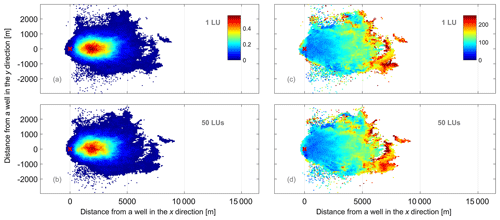Figure 11Probability of (a, b) and mean travel time required for (c, d) a particle leaving a given grid cell to reach a well accounting for a single randomly generated land-use map for all realizations (a, c) and for a different randomly generated land-use map for each realization (b, d).

For instance, results show that homogenized K fields perform more poorly in predicting the lowest concentrations (P90) than the highest ones (P50 and P10). From a NPS pollution management perspective, the accuracy of the higher concentrations exceeded by half of wells or even by just 10 % of wells is most critical, since they are more likely to exceed the MCL. The homogenized predictions are least accurate during the transition (breakthrough) period when concentrations in the vertically mixed sample obtained from a well are strongly controlled by travel time pdfs, which in turn are affected by the heterogeneity in the land use and aquifer dynamics.

7 Conclusions

A significant body of groundwater flow and transport literature has focused on upscaling flow and transport processes associated with industrial point source pollution. For accidental pollution with pollutants exceeding compliance levels by orders of magnitude, field research has shown that large uncertainties exist in predicting the fate of such contaminant plumes and the inability of upscaled methods to capture site-specific plume behavior. Stochastic methods have been used to characterize such large uncertainties. Here we explore the ability to which homogenized, effective representations of aquifer structure and landscape spatial variability in flow and transport simulations of NPS pollution are capable of accurately predicting pollution management metrics. We use three metrics typically of interest to NPS pollution management: travel time pdfs, stochastic capture zones, and stochastic breakthrough curves. We compare solutions of these metrics for a fully heterogeneous aquifer structure and landscape system with those of a homogenized, upscaled landscape system, those of a homogenized, upscaled aquifer system, and those of a completely homogenized aquifer and landscape system. Within the landscape system, we further distinguish between homogenizing recharge flux and homogenizing pollutant mass flux. The analysis is performed for a typical intensive, irrigated Mediterranean agricultural landscape of orchards, vineyards, and field crops overlying an alluvial aquifer system polluted with nitrate from fertilizer applications. Based on the simulation results presented, we make the following key conclusions.

• Land use, soil, and aquifer heterogeneity lead to large variability in groundwater travel paths, travel times, source location, and therefore well nitrate concentrations across a regional set of wells and, hence, significant uncertainty about pollution dynamics at any one well.

• The impact of continuous landscape pollutant loading on a typical high-capacity production well with the top of the screen at 100 m below the water table is first seen a couple of decades after pollution initiation but is not fully reflected across all wells of a region until 1 or 2 centuries later.

• With the capture zone of an individual well typically stretching across a diverse subset of land use in a region, the homogenization of the recharge and mass loading across the landscape to simulate NPS pollution management metrics can be appropriate, especially for simulating travel time pdfs and stochastic capture zones. In this case, nitrate variability between wells is much more affected by aquifer and soil heterogeneity than the heterogeneity in crop patterns across the landscape. This finding may not apply to cases where land-use units (fields, orchards) occupy a much larger area or many fields of one crop type are clustered, or for wells with small pumping rates and, hence, small capture zones – in those cases the variability in capture zone loading across an ensemble of wells may be ill-represented by a homogenized, regionally averaged recharge and nitrate mass loading.

• Homogenization of the aquifer hydraulic property significantly degrades travel time statistics as well as the stochastic delineation of the capture zone. Accounting for aquifer heterogeneity by utilizing an upscaled macrodispersion only slightly improves predictions of travel time pdfs or stochastic capture zones.

• During the transition period (20 to 170 years after pollution initialization), simulations using a homogenized representation of the aquifer structure provide aggregated concentration predictions, such as the concentration exceeded by half of wells, that are as much as a factor 2 different from predictions that fully represent aquifer heterogeneity.

• On the other hand, due to the strong effect of vertical groundwater mixing during the well pumping process and due to the continuity of NPS pollution, an upscaled, homogenized representation of aquifer heterogeneity using an effective hydraulic conductivity produces reliable and useful predictions for the concentration levels exceeded by half of the wells and even the higher concentrations exceeded by only 10 % of the wells, especially in the long term. These are the wells of most concern in NPS pollution of groundwater.

• Homogenized approaches may be most useful to predict whether long-term outcomes meet management goals across a regional ensemble of wells, but may be less accurate in specifying how quickly such goals may be achievable.

Future work is needed to further understand the role of crop-type clustering in landscape homogenization and the effect of interannual and seasonal loading variability on NPS pollution management metrics. More work is also needed to investigate other forms of partial or full homogenization of aquifer structure on prediction metrics considered here.

Appendix A: Well screen design

For each realization of the hydraulic conductivity field, three extraction wells are implemented. The pumping rate of each well is fixed to 3000 m3 d−1 and the top of the screen is fixed to 100 m. As in real settings, the length of this screen is dependent on the local aquifer properties in order to sustain the total extraction rate. Indeed, pumping effectively occurs through portions of wells located in highly conductive aquifer material. To simulate this local K dependence of the well screen length, we are using a rule of thumb stating that 10 cumulative feet (3.05 m) of gravel and sand have to be crossed for every 100 gallons per minute (545.1 m3 d−1) of extraction. The probability histogram (over all realizations) of the simulated screen lengths for each tested extraction rate is shown in Fig. A1 in the Appendix.

Appendix B: Impact of dispersion

Former studies highlighted the insensitivity of transport simulations to local-scale dispersivity (αi, where i indicates the transport direction) if aquifer heterogeneity is represented in a finely detailed manner by means of the transition probability method (TPROGS). This insensitivity is explained by the large macrodispersion caused by the well-represented facies-scale heterogeneity, which renders spreading from local dispersivity insignificant. As a result, fairly small values of αL are, in this setting, usually adopted. For instance, applied to their transport model (with a computational grid similar to the one used in our study) a grid-scale longitudinal dispersivity of 0.04 m, which appeared to have an insignificant impact on transport and resulting groundwater age distribution. Values of αL were chosen to fulfill the magnitude of dispersivity values reported at field sites of a scale similar to the computation cells (references in previously cited work). Here, we test the impact of much larger values of dispersivity (1.5 and 15.0 m) on breakthrough curves recorded at a well. The simulation setup is identical to the one described in the paper. Results are shown for a single realization of the hydraulic conductivity field.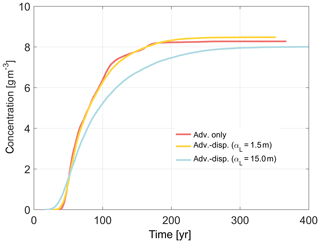Figure B1Breakthrough curve recorded at a well accounting for advection only (red curve), for advection and dispersion with a longitudinal dispersivity of 1.5 m (yellow curve), and for advection and dispersion with a longitudinal dispersivity of 15.0 m (blue curve). Transverse horizontal and transverse vertical dispersivities are always, respectively, 1∕10 and 1∕100 of the longitudinal dispersivity.

Our outputs display no significant impact on transport of a αL coefficient of 1.5 m. Increasing grid-scale dispersivity to 15 m leads to slightly earlier first travel times, later late travel times and lower contaminant levels observed at intermediate and late times (Fig. B1). Therefore, no implications can be expected when accounting exclusively for advection when grid dispersivity is lower than 1.5 m, as always used in previously cited studies.

Code availability

The online resources located in GitHub (see this link: https://github.com/chrishenri/NPS_stochastic_assessement; ) include the Matlab scripts necessary to reproduce the results.

Supplement

Author contributions

CVH and TH designed the Monte Carlo simulations, which were then run and post-processed by CVH. ED designed and ran the Hydrus simulations. All the authors contributed to the results analysis and to the writing of the article.

Competing interests

The authors declare that they have no conflict of interest.

Disclaimer

Any use of trade, firm, or product names is for descriptive purposes only and does not imply endorsement by the SWB.

Acknowledgements

The authors gratefully acknowledge the financial support through the California State Water Resources Control Board (SWB), agreement 15-062-250.

Financial support

This research has been supported by the California State Water Resources Control Board (grant no. 15-062-250).

Review statement

This paper was edited by Brian Berkowitz and reviewed by two anonymous referees.

References

Allen, R. G., Pereira, L. S., Raes, D., and Smith, M.: Crop evapotranspiration- Guidelines for computing crop water requirements, paper 56, FAO Irrigation and drainage, 1998. a, b

Baram, S., Couvreur, V., Harter, T., Read, M., Brown, P., Kandelous, M., Smart, D., and Hopmans., J.: Estimating Nitrate Leaching to Groundwater from Orchards: Comparing Crop Nitrogen Excess, Deep Vadose Zone Data-Driven Estimates, and HYDRUS Modeling, Vadose Zone J., 15, 1–13, https://doi.org/10.2136/vzj2016.07.0061, 2016. a

Barlow, P. M.,Leake, S. A., and Fienen, M. N.: Capture Versus Capture Zones: Clarifying Terminology Related to Sources of Water to Wells, Groundwater, 56, 694–704, 2018. a

Bastani, M. and Harter, T.: Effects of upscaling temporal resolution of groundwater flow and transport boundary conditions on the performance of nitrate-transport models at the regional management scale, Hydrogeol. J., accepted, 2020. a, b

Biggar, J. W. and Nielsen, D. R.: Spatial Variability of the Leaching Characteristics of a Field Soil, Water Resour. Res., 12, 78–84, 1976. a

Carle, S. F.: TProGS: Transition probability geostatistical software, Dep. of Land, Air and Water Resources, Univ. of Calif., Davis, 1999. a

Carle, S. F. and Fogg, G. E.: Transition probability-based indicator geostatistics, Math. Geol., 28, 453–477, 1996. a

Carle, S. F. and Fogg, G. E.: Modeling spatial variability with one-and multi-dimensional continuous Markov chains, Math. Geol., 29, 891–917, 1998. a

Conan, C., Bouraoui, F., Turpin, N., de Marsily, G., and Bidoglio, G.: Modeling Flow and Nitrate Fate at Catchment Scale in Brittany (France), J. Environ. Qual., 32, 2026–2032, 2003. a

Cvetkovic, V., Shapiro, A. M., and Dagan, G.: A solute flux approach to transport in heterogeneous formations: 2. Uncertainty analysis, Water Resour. Res., 28, 1377–1388, 1992. a, b, c

Dagan, G.: Solute transport in heterogeneous porous formations, J. Fluid Mech., 145, 151–177, 1984. a, b

Dagan, G.: Transport in heterogeneous porous formations: Spatial moments, ergodicity, and effective dispersion, Water Resour. Res., 26, 1281–1290, https://doi.org/10.1029/WR026i006p01281, 1990. a, b, c, d

Dagan, G. and Nguyen, V.: A comparison of travel time and concentration approaches to modeling transport by groundwater, J. Contam. Hydrol., 4, 79–91, 1989. a, b

de Barros, F. P. J. and Nowak, W.: On the link between contaminant source release conditions and plume prediction uncertainty, J. Contam. Hydrol., 116, 24–34, https://doi.org/10.1016/j.jconhyd.2010.05.004, 2010. a

de Barros, F. P. J. and Rubin, Y.: A risk-driven approach for subsurface site characterization, Water Resour. Res., 44, W01414, https://doi.org/10.1029/2007WR006081, 2008. a

Diamantopoulos, E. and Durner, W.: Dynamic Nonequilibrium of Water Flow in Porous Media: A Review, Vadose Zone J., 11, https://doi.org/10.2136/vzj2011.0197, 2012. a

Faunt, C. C. (Ed.): Groundwater Availability of the Central Valley Aquifer, California: U.S. Geological Survey Professional Paper 1766, 225 pp., 2009. a

Feddes, R. A., Kowalik, P. J., and Zaradny, H.: Simulation of field water use and crop yield, Simulation monographs, Pudoc, Wageningen, the Netherlands, 1978. a

Fernàndez-Garcia, D., Illangasekare, T. H., and Rajaram, H.: Differences in the scale-dependence of dispersivity estimated from temporal and spatial moments in chemically and physically heterogeneous porous media, Adv. Water Res., 28, 745–759, 2005. a

Fleckenstein, J. and Fogg, G.: Efficient upscaling of hydraulic conductivity in heterogeneous alluvial aquifers, Hydrogeol. J., 16, 1239, https://doi.org/10.1007/s10040-008-0312-3, 2008. a

Franzetti, S. and Guadagnini, A.: Probabilistic estimation of well catchments in heterogeneous aquifers, J. Hydrol., 174, 149–171, 1996. a

Frind, E. O., Molson, J. W., Schirmer, M., and Guiguer, N.: Dissolution and mass transfer of multiple organics under field conditions: The Borden emplaced source, Water Resour. Res., 35, 683–694, https://doi.org/10.1029/1998WR900064, 1999. a

Gelhar, L. W.: Stochastic subsurface hydrology: Englewood Cliffs, New Jersey, Prentice-Hall, 390 pp., 1993. a

Gibbons, R. D.: Statistical Methods for Groundwater Monitoring, Wiley, 2 edition, Hoboken, New Jersey, 1994. a

Hansen, B., Dalgaard, T., Thorling, L., Sørensen, B., and Erlandsen, M.: Regional analysis of groundwater nitrate concentrations and trends in Denmark in regard to agricultural influence, Biogeosciences, 9, 3277–3286, https://doi.org/10.5194/bg-9-3277-2012, 2012. a

Harbaugh, A., Banta, E., Hill, M., and McDonald, M.: MODFLOW 2000 the US Geological Survey Modular ground-water model-user guide to modularization concepts and the ground-water flow process, Open File 00-92, Rep. U.S. Geol. Surv., 121 pp., 2000. a

Harter, T., Lund, J. R., Darby, J., Fogg, G. E., Howitt, R., Jessoe, K. K., Pettygrove, G. S., Quinn, J. F., Viers, J. H., Boyle, D. B., Canada, H. E., DeLaMora, N., Dzurella, K. N., Fryjoff-Hung, A., Hollander, A. D., Honeycutt, K. L., Jenkins, M. W., Jensen, V. B., King, A. M., Kourakos, G., Liptzin, D., Lopez, E. M., Mayzelle, M. M., McNally, A., Medellin-Azuara, J., and Rosenstock, T. S.: Addressing Nitrate in California's Drinking Water with a Focus on Tulare Lake Basin and Salinas Valley Groundwater, Report, 78 pp., Center for Watershed Sciences, University of California, Davis for the State Water Resources Control Board Report to the Legislature, 2012. a, b

Harter, T., Dzurella, K., Kourakos, G., Hollander, A., Bell, A., Santos, N., Hart, Q.,King, A., Quinn, J., Lampinen, G., Liptzin, D., Rosenstock, T., Zhang, M., Pettygrove, G. S., and Tomich, T.: Nitrogen Fertilizer Loading to Groundwater in the Central Valley. Final Report to the Fertilizer Research Education Program, Tech. Rep. Projects 11‐0301 and 15‐0454, California Department of Food and Agriculture and University of California Davis, 2017. a, b

Henri, C. V.: Stochastic Assessment for Non-Point Source Contamination of Heterogeneous Aquifer: Codes, and Instructions for Inputs and Outputs, available at: https://github.com/chrishenri/NPS_stochastic_assessement, last access: 16 April 2019. a

Henri, C. V. and Fernàndez-Garcia, D.: Toward efficiency in heterogeneous multispecies reactive transport modeling: A particle-tracking solution for first-order network reactions, Water Resour. Res., 50, 7206–7230, 2014. a

Henri, C. V. and Fernàndez-Garcia, D.: A random walk solution for modeling solute transport with network reactions and multi-rate mass transfer in heterogeneous systems: Impact of biofilms, Adv. Water Resour., 86, 119–132, https://doi.org/10.1016/j.advwatres.2015.09.028, 2015. a

Henri, C. V. and Harter, T.: Stochastic assessment of nonpoint source contamination: Joint impact of aquifer heterogeneity and well characteristics on management metrics, Water Resour. Res., 55, 6773–6794, https://doi.org/10.1029/2018WR024230, 2019. a, b, c, d, e, f, g

Henri, C. V., Fernàndez-Garcia, D., and de Barros, F. P. J.: Assessing the joint impact of DNAPL source-zone behavior and degradation products on the probabilistic characterization of human health risk, Adv. Water Resour., 88, 124–138, 2016. a, b

Hillel, D.: Fundamental of Soil Physics, Academic Press, New York, 1980. a

Horn, J. E. and Harter, T.: Domestic Well Capture Zone and Influence of the Gravel Pack Length, Groundwater, 47, 277–286, https://doi.org/10.1111/j.1745-6584.2008.00521.x, 2009. a, b

Hua, Z. and Harter, T.: Nonpoint source solute transport normal to aquifer bedding in heterogeneous, Markov chain random fields, Water Resour. Res., 42, W06403, https://doi.org/10.1029/2004WR003808, 2006. a

Jordan, T. E., Correll, D. L., and Weller, D. E.: Relating nutrient discharges from watersheds to land use and streamflow variability, Water Resour. Res., 33, 2579–2590, https://doi.org/10.1029/97WR02005, 1997. a

Kladivko, E. J., Frankenberger, J. R., Jaynes, D. B., Meeka, D. W., Jenkinson, B. J., and Fausey., N. R.: Nitrate Leaching to Subsurface Drains as Affected by Drain Spacing and Changes in Crop Production System Contribution of the Indiana Agric. Research Programs, J. Environ. Qual., 33, 1803–1813, 2004. a

Koh, E.-H., Lee, E., Kaown, D., Green, C. T., Koh, D.-C., Lee, K.-K., and Lee, S. H.: Comparison of groundwater age models for assessing nitrate loading, transport pathways, and management options in a complex aquifer system, Hydrol. Process., 32, 923–938, https://doi.org/10.1002/hyp.11465, 2018. a, b

Kourakos, G., Klein, F., Cortis, A., and Harter, T.: A groundwater nonpoint source pollution modeling framework to evaluate long-term dynamics of pollutant exceedance probabilities in wells and other discharge locations, Water Resour. Res., 48, https://doi.org/10.1029/2011WR010813, 2012. a

LaBolle, E. M.: Theory and simulation of diffusion processes in porous media, PhD dissertation, Univ. of Calif., Davis, CA, 202 pp., 1999. a, b

LaBolle, E. M. and Fogg, G. E.: Role of molecular diffusion in contaminant migration and recovery in an alluvial aquifer system, Transp. Porous Media, 42, 155–179, 2001. a, b

Li, L. and Graham, W.: Stochastic analysis of solute transport in heterogeneous aquifers subject to spatially random recharge, J. Hydrol., 206, 16–38, 1998. a, b

Loague, K. and Corwin, D. L.: Regional-scale assessment of non-point sourcegroundwater contamination, Hydrol. Process., 12, 957–965, 1998. a

Logsdon, S. D., Kaspar, T. C., Meek, D. W., and Prueger, J. H.: Nitrate Leaching as Influenced by Cover Crops in Large Soil Monoliths, Agron. J., 94, 807–814, https://doi.org/10.2134/agronj2002.8070, 2002. a

Nielsen, D. R., Biggar, J. W., and Erh, K. T.: Spatial variability of field-measured soil-water properties, Hilgardia, 42, 215–259, 1973. a

Nolan, B. T., Hitt, K. J., and Ruddy, B. C.: Probability of Nitrate Contamination of Recently Recharged Groundwaters in the Conterminous United States, Environ. Sci. Technol., 36, 2138–2145, 2002. a

Nolan, B. T., Green, C. T., Juckem, P. F., Liao, L., and Reddy, J. E.: Metamodeling and mapping of nitrate flux in the unsaturated zone and groundwater, Wisconsin, USA, J. Hydrol., 559, 428–441, https://doi.org/10.1016/j.jhydrol.2018.02.029, 2018. a

Perfect, E., Sukop, M. C., and Haszler, G. R.: Prediction of Dispersivity for Undisturbed Soil Columns from Water Retention Parameters, Soil Sci. Soc. Am. J., 66, 696–701, 2002. a

Perrone, D. and Jasechko, S.: Deeper well drilling an unsustainable stopgap to groundwater depletion, Nature Sustainability, 2, 773–782, https://doi.org/10.1038/s41893-019-0325-z, 2019. a

Rajaram, H.: Perturbation theories for the estimation of macrodispersivities in heterogeneous aquifers, in: Stochastic methods in subsurface contamination hydrology, edited by: Govindaraju, R. S., 13–62, 2002. a

Ransom, K., King, A., and Harter, T.: Identifying sources of groundwater nitrate contamination in a large alluvial groundwater basin with highly diversified intensive agricultural production, J. Contam. Hydrol., 151, 140–154, https://doi.org/10.1016/j.jconhyd.2013.05.008, 2013. a

Ritter, W. F. and Shirmohammadi, A.: Agricultural Nonpoint Source Pollution: Watershed Management and Hydrology, CRC Press, Boca Raton, 2000. a

Riva, M., Guadagnini, A., and Ballio, F.: Time-related capture zones for radial flow in two dimensional randomly heterogeneous media, Stoch. Env. Res. Risk A., 13, 217–230, 1999. a

Rockström, J., Steffen, W., Noone, K., Persson, Å., Chapin III, F. S., Lambin, E. F., Lenton, T. M., Scheffer, M., Folke, C., Schellnhuber, H. J., Nykvist, B., de Wit, C. A., Hughes, T., van der Leeuw, S., Rodhe, H., Sörlin, S., Snyder, P. K., Costanza, R., Svedin, U., Falkenmark, M., Karlberg, L., Corell, R. W., Fabry, V. J., Hansen, J., Walker, B., Liverman, D., Richardson, K., Crutzen, P., and Foley, J. A.: A safe operating space for humanity, Nature, 461, 472–475, 2009. a, b

Rubin, Y.: Applied Stochastic Hydrogeology, Oxford Univ. Press, Oxford, 2003. a, b

Rushton, K. and Redshaw, S.: Seepage and groundwater flow – Numerical analysis by analogue and digital methods, John Wiley and Sons, New York, 1979. a

Salamon, P., Fernandez-Garcia, D., and Gomez-Hernandez, J. J.: A review and numerical assessment of the random walk particle tracking method, J. Contam. Hydrol., 97, 277–305, 2006. a

Scaap, M. G., Nemes, A., and van Genuchten, M. T.: Comparison of Models for indirect estimation of water retention and available water in surface soils, Vadose Zone J., 3, 1455–1463, 2004. a

Sisson, J. B. and Wierenga, P. J.: Spatial Variability of Steady-State Infiltration Rates as a Stochastic Process, Soil Sci. Soc. Am. J., 45, 699–704, 1981. a

Stauffer, F., Attinger, S., Zimmermann, S., and Kinzelbach, W.: Uncertainty estimation of well catchments in heterogeneous aquifers, Water Resour. Res., 38, 1238, https://doi.org/10.1029/2001WR000819, 2002. a

Survey, U. S. G., Burton, C. A., Shelton, J. L., and Belitz, K.: Status and understanding of groundwater quality in the two southern San Joaquin Valley study units, 2005–2006 – California GAMA Priority Basin Project, Tech. rep., USGS, Reston, VA, https://doi.org/10.3133/sir20115218, 2012.  a

Survey, U. S. G., Shelton, J. L., Fram, M. S., Belitz, K., and Jurgens, B. C.: Status and understanding of groundwater quality in the Madera, Chowchilla Study Unit, 2008: California GAMA Priority Basin Project, Tech. rep., USGS, Reston, VA, https://doi.org/10.3133/sir20125094, 2013. a

United States Department of Agriculture: irrigation guide: Natural Resources Conservation Service, National engineering handbook part 652, USDA, available at: https://directives.sc.egov.usda.gov/viewerFS.aspx?hid=21431 (last access: 14 April 2004), 1997. a

van Genuchten, M.: A closed form equation for predicting the hydraulic conductivity of unsaturated soils, Soil Sci. Soc. Am. J., 44, 892–898, 1980. a

Varljen, M. D. and Shafer, J. M.: Assessment of uncertainty in time-related capture zones using conditional simulation of hydraulic conductivity, Ground Water, 29, 737–748, 1991. a

Vereecken, H., Kamai, T., Harter, T., Kasteel, R., Hopmans, J., and Vanderborght, J.: Explaining soil moisture variability as a function of mean soil moisture: A stochastic unsaturated flow perspective, Geophys. Res. Lett., 34, L22402, https://doi.org/10.1029/2007GL031813, 2007. a

Šimunek, J., van Genuchten, M., and Šejna, M.: Recent developments and applications of the HYDRUS computer software packages, Vadose Zone J., 15, 1–25, https://doi.org/10.2136/vzj2016.04.0033, 2016. a

Weissmann, G. S. and Fogg, G. E.: Multi-scale alluvial fan hetero-geneity modeled with transition probability geostatistics in a sequence stratigraphic framework, J. Hydrol., 226, 48–65, 1999a. a

Weissmann, G. S. and Fogg, G. E.: Three-dimensional hydrofacies modeling based on soil surveys and transition probability geostatistics, Water Resour. Res., 35, 1761–1770, 1999b. a

Weissmann, G. S., Zhang, Y., LaBolle, E. M., and Fogg, G. E.: Dispersion of groundwater age in an alluvial aquifer system, Water Resour. Res., 38, 1198, https://doi.org/10.1029/2001WR000907, 2002. a, b, c, d, e, f, g

Zektser, I. S. and Everett, L. G.: Groundwater resources of the world and their use, IHP-VI, series on groundwater 6, UNESCO, 2004. a